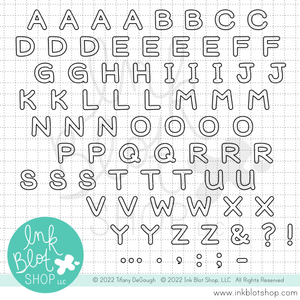Welcome!
Welcome!Ink Blot Shop LLC

# Outline Alpha :: 4x8 Clear Stamp Set

Regular Price \$17.99 \$17.99

Outline Alpha is a 4" x 8" alphabet stamp set that includes 84 individual stamps.

For size reference, the letters are approximately 3/8" tall.

Outline Alpha includes the following letters, punctuation, and images: A (x4), B (x2), C (x2), D (x3), E (x4), F (x2), G (x2), H (x2), I (x3), I (x3), J (x2), K (x2), L (x3), M (x3), N (x3), O (x4), P (x2), Q (x2), R (x3), S (x3), T (x3), U (x2), V (x2), W (x2), X (x2), Y (x2), Z (x2), ampersand (x1), period (x3), comma (x2), exclamation mark (x2), question mark (x1), semicolon (x1), colon (x2), ellipsis (x2), and a hyphen/dash (x1).